# OECD Meat Consumption Data Analysis 6 - POULTRY Consumption is positively correlated with GDP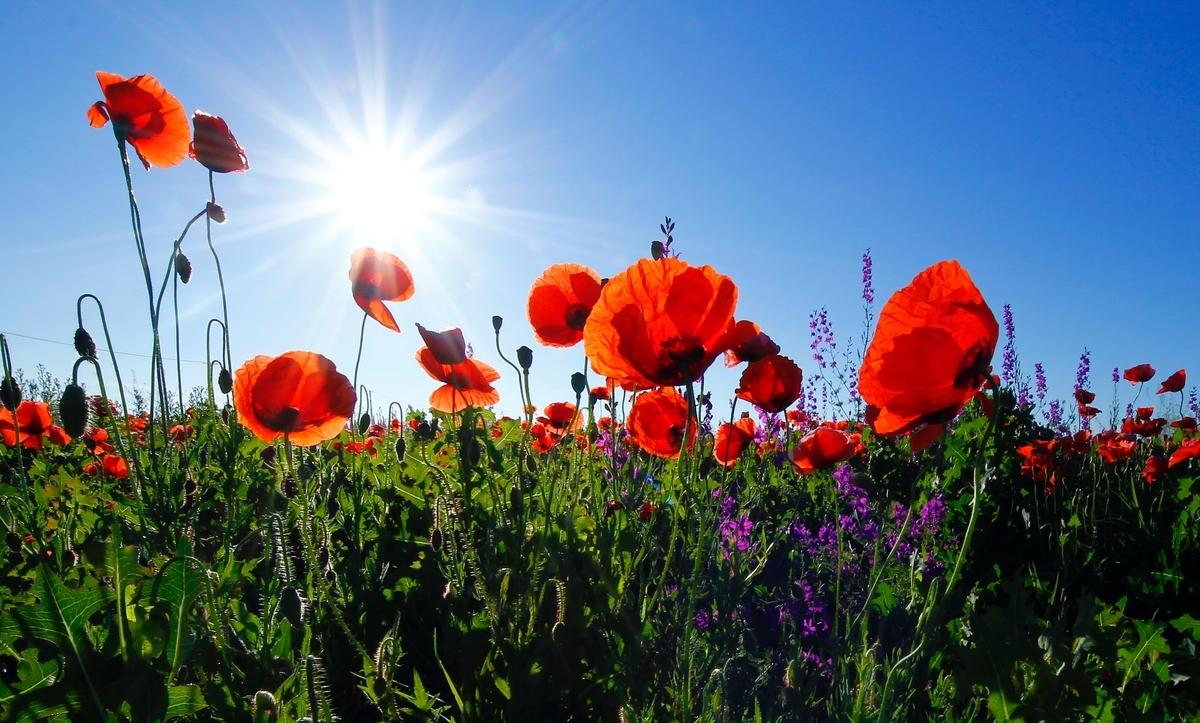Photo by corina ardeleanu on Unsplash

This post is following of above post.

I have GDP data like below CSV file.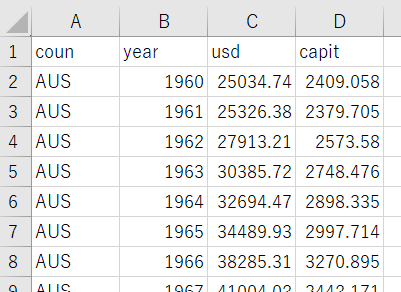So, let's combine this GDP data and Meat Consumption data.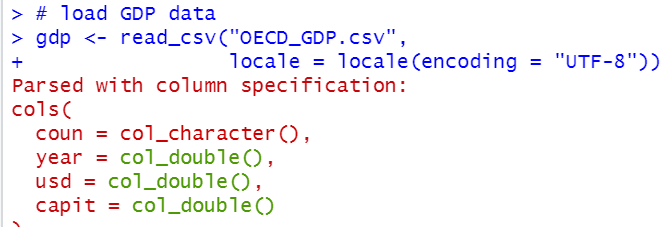Next, I use inner_join() function to combine df2 dataframe and gdp dataframe.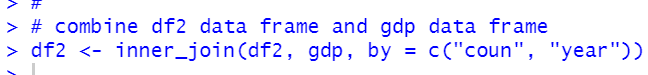Let's see the result.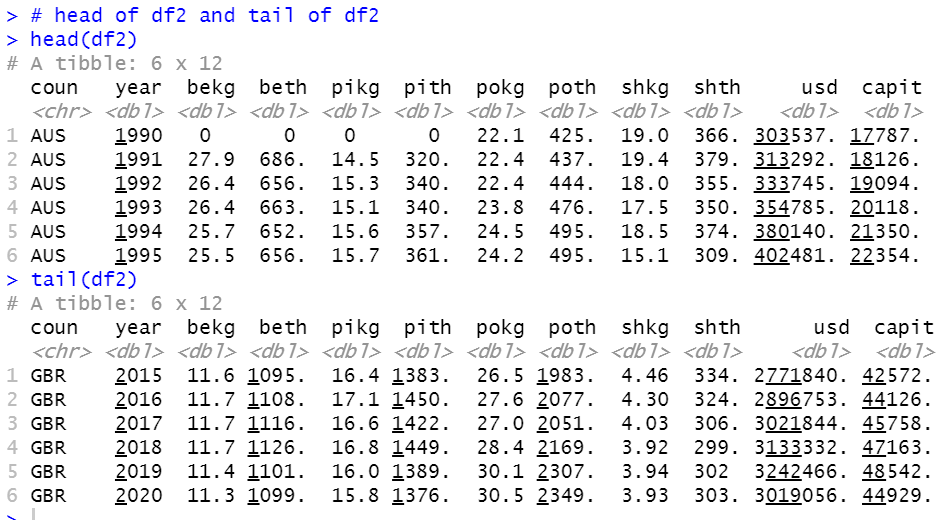usd is gross GDP value, capit is per capita GDP.

summary if df2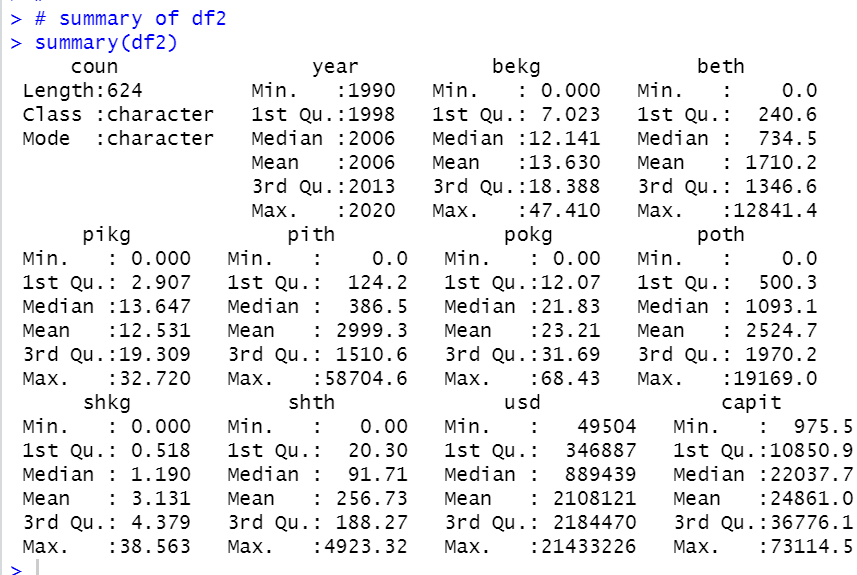We see year range is changed. 1990 ~ 2020.

Now, let's see capit and 4 KG_CAP data.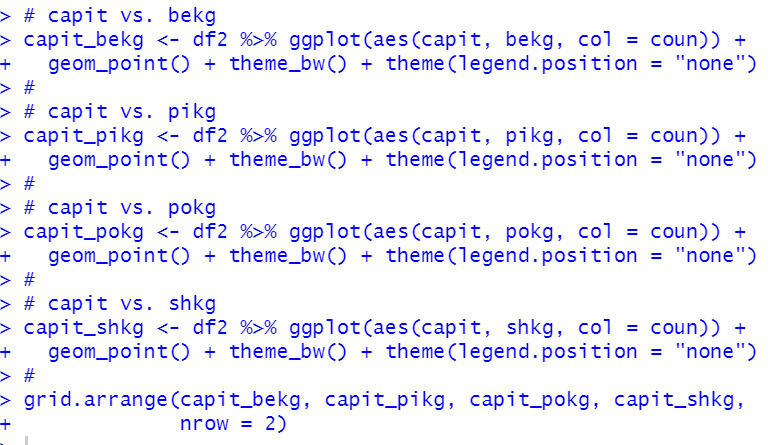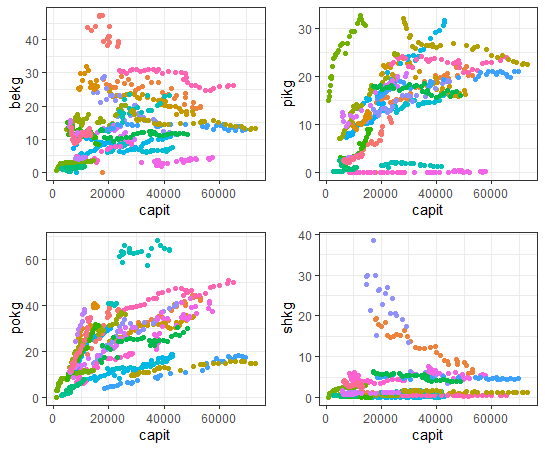I see shkg is not so much correlated with capit.

Let's see correlation with capit and 4 KG_CAPs by country.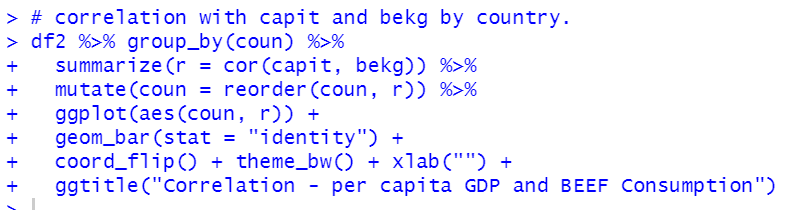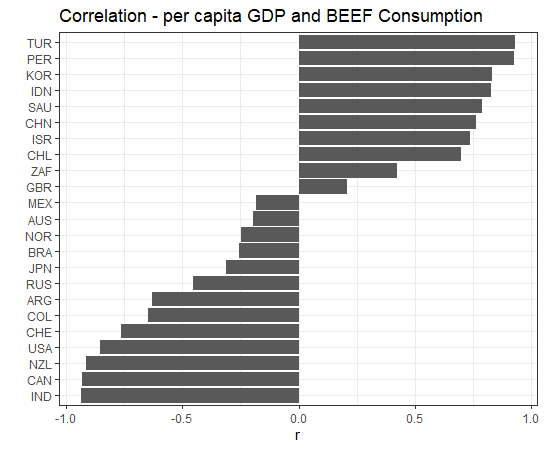We see some countries have negative correlations and some contries have positive correlations.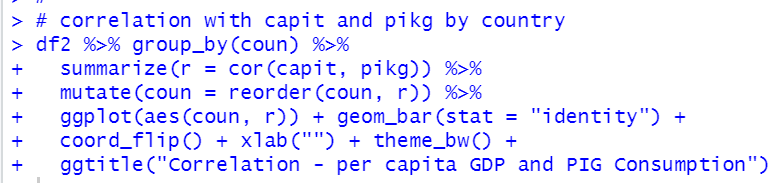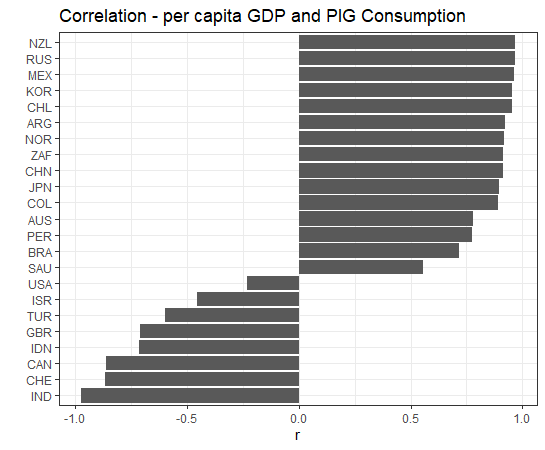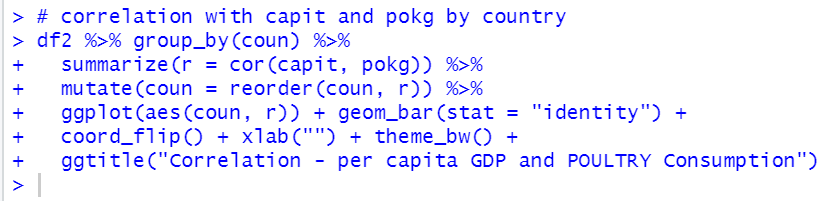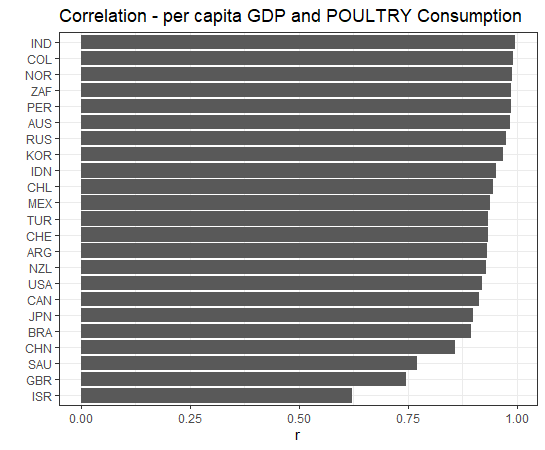All countires have positive correlations with POULTRY.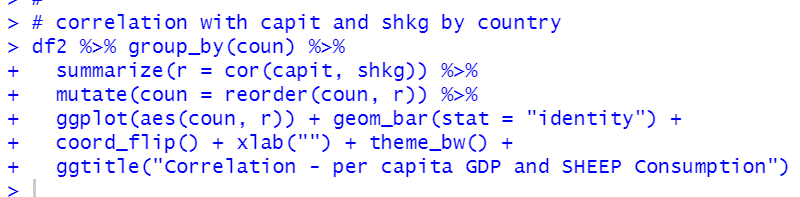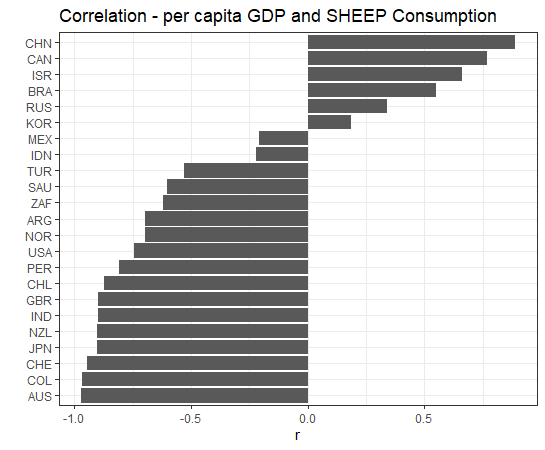We see some countries have negative correlation while some countries have positive correlations.

per capita POULTRY Consumption and per capita GDP are positively correlated in all countries.

That's it. Thank you!

Next post is